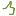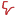No tags

Al Salaam,

Numerology – The Ancient Science of Calculating Numbers by Letters or Glyphs and its relation to the planets and stars.

The Sister Science being Astrology/Astronomy which is the calculation of degrees in each constellation and its relation to planets and stars.

The Science of MR
Moorish Mathematics 0-9 Sacred Geometry & 13Numerical Chart 1-9                                                       M = 4                                                                               R = 9    4+9 = 13 = 4

Numerical Value = {M = 13 LE TTER= 4} & {R = 18 LE TTER = 9}

4 + 9 = 13 = 4
MR = 13 = 4 = Pyramid

MR is the translation for Pyramid in Ancient Moorish/Khemitian Language

The Numerical Value of Lights Green & Red
{Green = 7 + 9 + 5 + 5 + 5 = 31 = 4} G =7 R=9 E=5 E=5 N=5

{Red = 9+5+4 = 18 = 9} R=9 E=5 D=4
4 + 9 = 13 = 4

MR = Green & Red = 13 = Pyramid

4th Month = April & 9th Month = September (Contemporary/Modern 12 Month)

March 20-April 19 = Portal = Spring Equinox = March 20
September 22 – October 22 = Portal = Autumn Equinox = Sept 22
Aries = March 21-April 19 & Libra = October 22-September 23
Aries = Mars & Libra = Venus
Aries = Red & Libra = Green
Moreover 9 is Red & 4 is Green. In Numerical the M is 4 & R is 9.
Aries reflection is Libra, They are the 1st & 7th Sign

From these small degrees of common knowledge we can see that “MR” not only equals the energy of 13/4, moreover it holds the energy of the EQUINOX  (Aries & Libra) and lights of GREEN & RED. How many National Flags are Green & Red around the World? How many Cultures around the world wear Green & Red clothing? Leaves turn Green to Red Spring to Autumn

4th Zodiac = Cancer & 9th Zodiac = Sagittarius
Cancer = “June 21” – July 22 = Portal = Summer Solstice
Sagittarius = November 22 – “December 21” = Portal = Winter Solstice
Cancer reflection is Capricorn starting at December 22

From these small degrees of common knowledge we can see that “MR” holds the energy of the SOLSTICE  (Cancer & Sagittarius) from natural zodiac law 12

52 Weeks in a Year
26 Weeks From Equinox to Equinox
26 Weeks From Solstice to Solstice
13 Weeks between Equinox to Solstice & Solstice to Equinox

{Spring Equinox -March 20/1 – Summer Solstice – June 21} = 13 Weeks
{ Summer Solstice – June 21 – Autumn Equinox – September 23} = 13 Weeks
{Autumn Equinox – September 23 – Winter Solstice – December 21} = 13 Weeks
{Winter Solstice – December 21 – Spring Equinox – March 20/1} = 13 Weeks

From these small degrees of common knowledge we can see that “MR” holds the energy of the SOLSTICE & EQUINOX by the nature of 4/9

4th Month = June & 9th Month = November (Moorish/Nature)
June/July = Cancer = Water Element = Beginning Summer Season
November/December = Sagittarius = Fire Element =  Ending Autumn Season
Cancer = Moon = Water = Silver
Sagittarius = Jupiter = Fire = Tin
4 = M = Water /Mother/Mu
9 = R = Fire/Ra/SunRe/Heru
2 Equinox & 2 Solstice = 4 Portals / Seasons/ Elements/ Quadrants
Seasons = Spring Summer Autumn Winter
Elements = Fire Earth Air Water

From these small degrees of common sense we can see that “MR” is WATER & FIRE , it is The EQUINOX & SOLSTICE meaning it is the YIN & YANG

MR is the Balance & Harmony of The Universe Measures (Equinox)
Moor/Maur/Mahārāja/Marza/Martinez/Ramirez/Markia/Master/Marcella/Markovich/Markice/Maria/Marzetter/Markov/Mario/Marcel/Marquez/Marino/Maraga/Marco/Martin/Mayorga/Morro/Mary/Marrieti/Mariah/Marciszewski/Marocco/Mara/Mero/Martina/  Marsala/Morochuco/Morehart/Mori/Maurus/Moore/Mórdha/Muir//Maurice/Mura/Morris/ Markus/Mark/Murgo/Mother/Matter/Miracle/Mermaid/Moral/Immortal/Mural etc is MR

Moor denotes Water(Ocean, Sea, Lake) & Earth ( Land , Pyramid)
Moor also denotes Expansion & Knowledge & Light (Fire)
Moor is also to connect , link , tie together (Mooring)
Moorish denotes Relation to Earth Water Fire which produces Air
Moors are then Indigenous by Birthright because they connect themselves to the Water & Earth & Fire & Air

Moors Carry Love and Empty Hate
(Moors Mooring through Portal Autumn Equinox Libra through Portal Spring Equinox Aries)
Moors Carry Light & Empty Darkness
(Moors Mooring through Portal Winter Solstice to Portal Summer Solstice)
Meaning Moors Hold Light to All Mystery Standing Square 360 *
Square = 4 Angles * 90 = 360 degrees (4,9) = 13 = 4
Triangle 3 Angles = 180 degrees (3,6) = 9
Circle = 360 degrees (9)
rectangle = 360 degrees (9)

Solstice is Latin for Sun Stands
Moors are the Solstice, The Walking Souls
Equinox is Equal Night & Day
Moors are the Equinox, The Knights & Deys = The Eq ALs
Making Moors The Nobles by Birthright

Knowing Your Equinox Degrees  is knowing you are Equal to all Souls on Earth. Common Law says this is common sense. With the combined Degrees of the Solstice your Nationality (Status) Equates your Jurisdiction

If you study Jurisprudence You Know the Positive Science this Math is Showing ! This Math is Absolute & Ultimate

These are the Solstice & Equinox Degrees, i wonder how many of the “Master & PHD” students know these degrees of common sense ?

Likes(5)Dislikes(0)